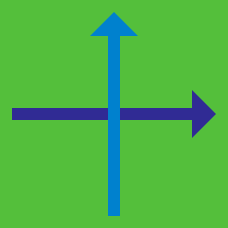Geometry

Coordinate Geometry Warmup

$(4, 5)$ is the midpoint between $(2, 1)$ and $(x, y).$ What is the value of $xy?$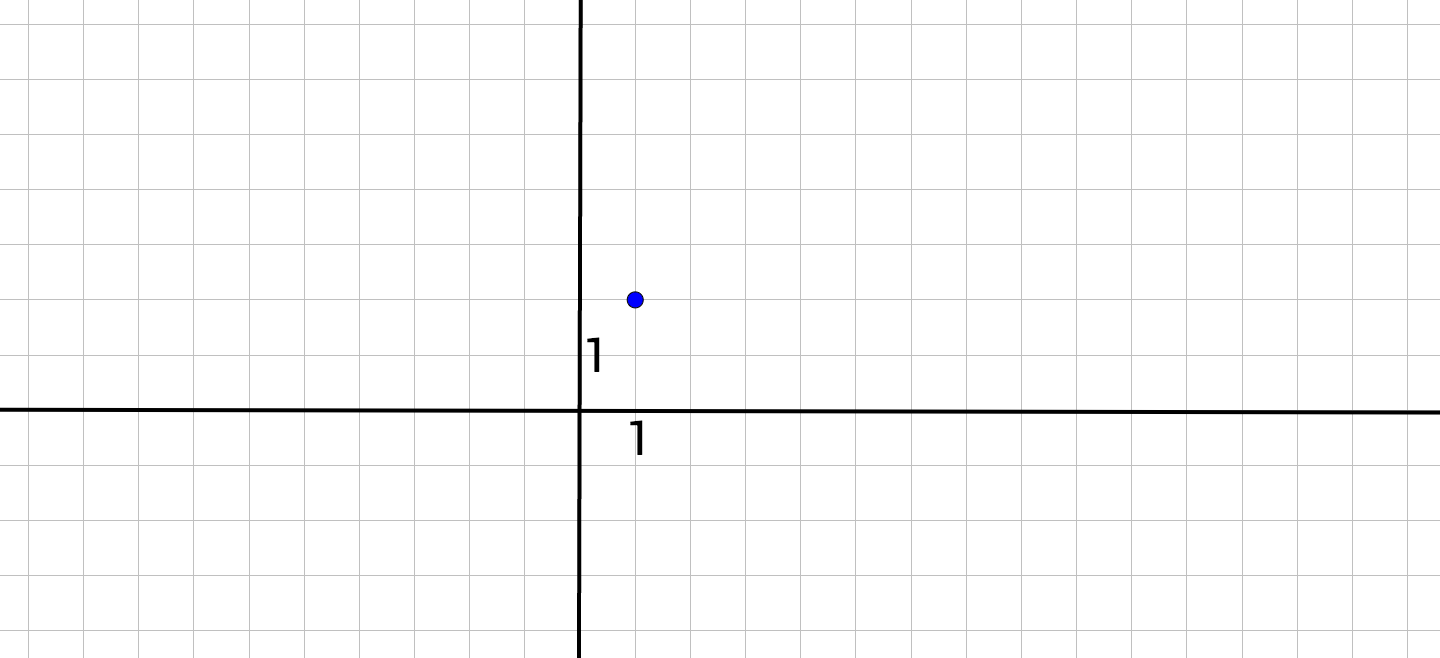A bug sitting at the point $(1, 2)$ moves 5 units vertically (parallel to the $y$-axis) and 1 units horizontally (parallel to the $x$-axis). Which ordered pair gives potential coordinates for the bug's new location?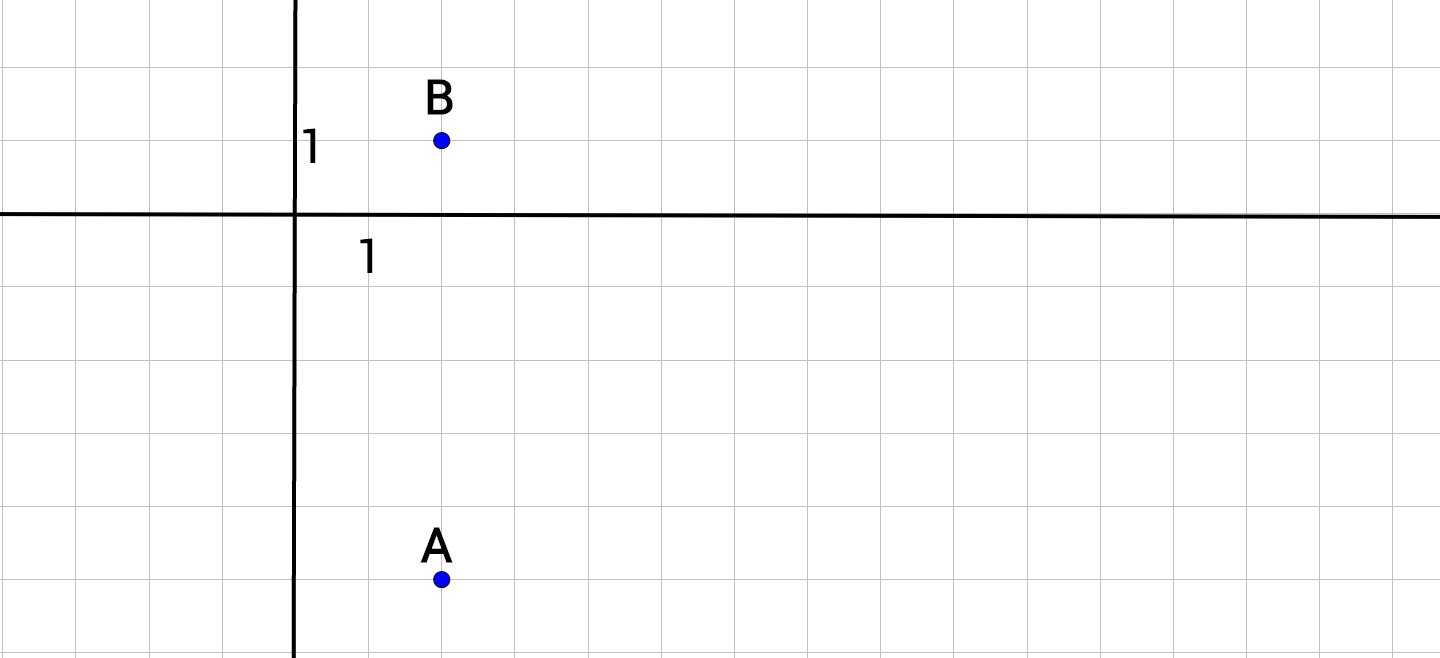The points $A, B, C,$ and $D$ form a rectangle that is twice as tall as it is wide. Given the coordinates of two of the points $A = (2, -5),$ and $B = (2, 1),$ where might another point of the rectangle ($C$ or $D$) be located?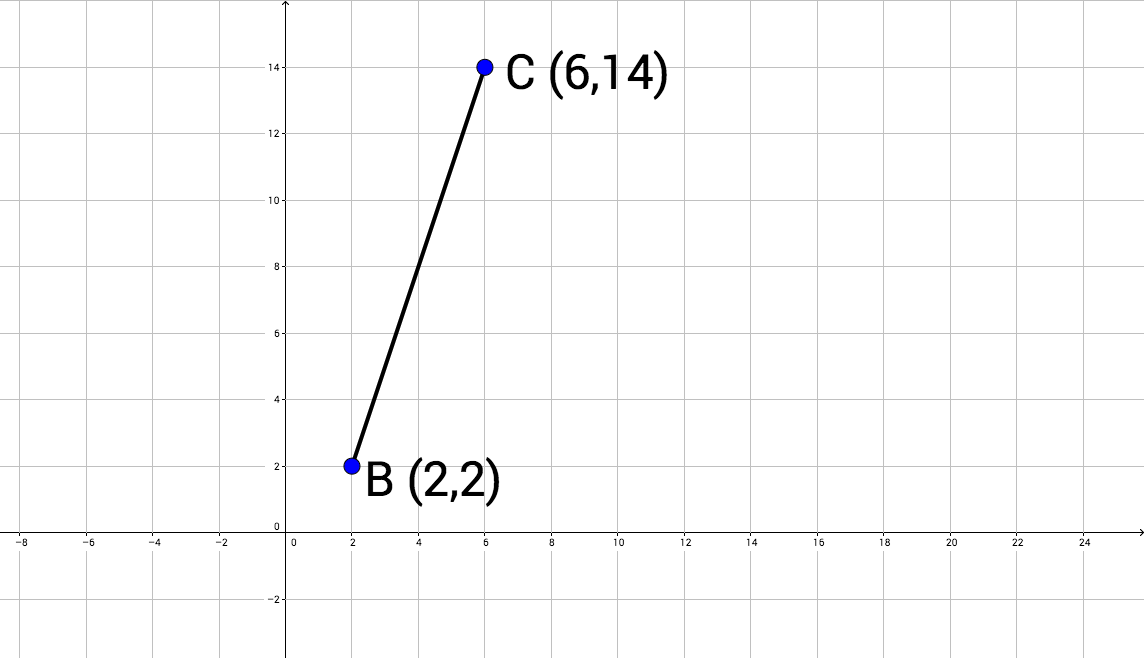$Q = (x, y),$ is a point on line segment $BC$ such that, comparing the lengths, $BQ:QC = 1:3.$ What is the value of $x + y$ if $B$ is the point (2, 2), and $C,$ the point (6, 14).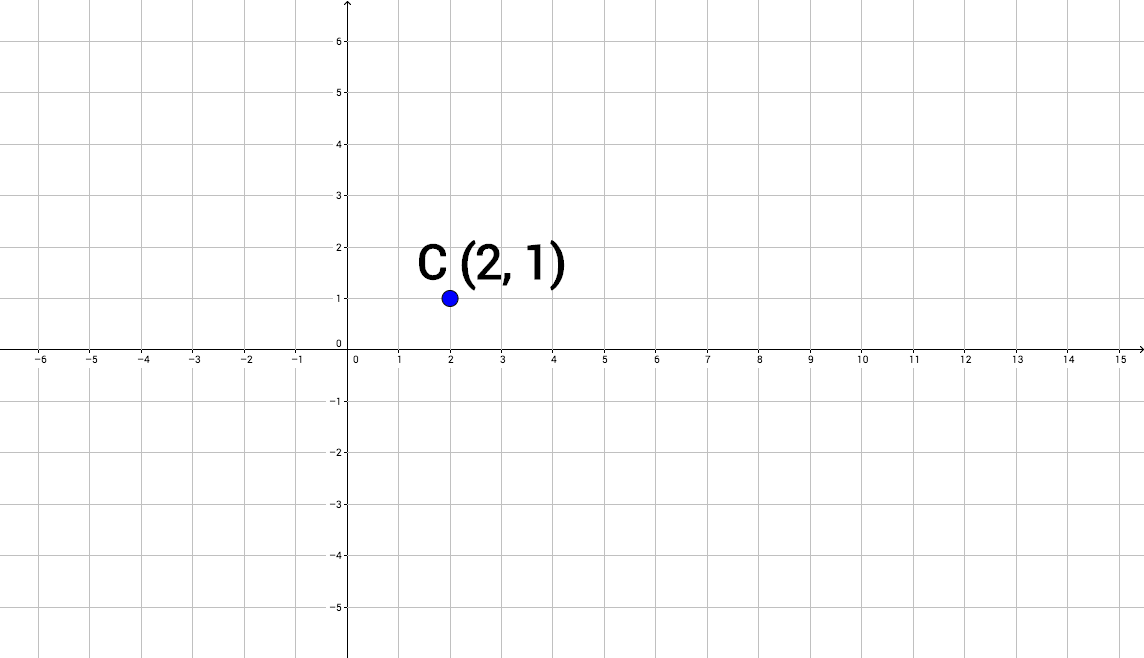Circle $C$ has a center at $(2, 1)$ with a radius of 5. Which point could lie on the circle?

×